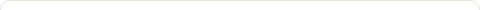# Photometry (Part 1)

Posted on: December 7, 2008

Photometry is a branch field of astrophysics which learned the quantity, quality, and the direction of the electromagnetic radiation from the sky’s objects. “Photo” in photometry which means “visual light” was used because the observation was used to limited in visual light.

Photometry was based on our knowledge about radiation law. We hypothesize that the astronomical objects have characteristics of a hypothetical black body.

The characteristics of the black body are:

1. when thermal equilibrium is achieved, the object’s temperature is a function of how many energy it absorbs per second
2. a black body doesn’t emit radiation in all wavelength in same intensity (some emits more radiation in blue region wavelength, and the way around. The wavelength which it emits most will determine its color).

The wavelength its emits most (λmaks) by a black body which temperature is T Kelvin is :

λmaks = 0,2898/ T …………………….. (eq. 1)

(λmaks expressed in cm and T in Kelvin)

The equation 1 is called Wien’s rule.

An example of implementing the Wien’s rule :(Warning : be clear that λmaks doesn’t mean the maximum wavelength but it means the wavelength that a body emits in the biggest intensity)

The total energy per time emitted by a black body per its surface area is called emitted energy flux. The value of the emitted energy flux from a black body with a surface temperatur T Kelvin is :

F = σT4 …………………….. (eq. 2)

(σ : Stefan-Boltzman constant: 5,67 x 10^-8 Watt/m2K4)

The total energy per unit time (= Power) that’s emitted by a black body with the surface temperature T Kelvin and surface area A is known as Luminosity. Its value (L) can be calculated by equation below:

L = A σT4 …………………….. (eq. 3)

For stars, we can assume it’s a perfect sphere. So, its surface area (A) is 4πR2 ; with R express star’s radius. So, a star’s luminosity (L) is equal to :

L = 4πR2 σT4 …………………….. (eq. 4)

he black body emits its radiation to all direction. We can assume the radiation pass through a sphere surface with a radius d in same energy flux (E).

E = L/(4πd2) …………………….. (eq. 5)

This amount of flux is received by an observer from a distance d from the black body. So, this flux is usually called received energy flux or brightness. (Warning : differ between E and F).

The equation above is often termed as the inverse square law for brightness (E) because this equation shows that brightness is inversely proportional to the square of its distance (d). So, the farther the distance, the less bright it is.

Review Questions:

1. From an observation result, we know that an area of 1 cm2 in Earth’s outer atmosphere received Sun’s energy with intensity of 1,37 x 106 erg/cm2/s. If we know that the distance between Sun and Earth is 150 million kilometres, determine the Sun’s luminosity.
2. Calculate the intensity of Sun’s radiation received by Saturn’s surface if we know that the distance between Saturn and Sun is 9,5 Astronomical Units (use the information from number 1)?
3. The luminosity of a star is 100 times larger than Sun is, but the temperature of the star is only half of Sun’s temperature. Calculate the radius of the star expressed in Sun’s radius unit?
4. Define the term Luminosity and Brightness using your own words
5. Calculate the wavelength of maximum intensity radiation of a star which temperature is 10.000 Kelvin.
(source : Dr. Djoni N. Dawanas)
(translated from : belajar Astronomy).

### 2 Responses to "Photometry (Part 1)"if you want to read the indonesian versio, go to :
http://hansgunawan-astronomy.blogspot.com/2008/10/pengenalan-fotometri-bagian-1.htmlYou are encourage to try the review question for reviewing things you have learned.### Pagesasad123 on Trivia Questionleaflonnne on Trivia Questionleaflonnne on Trivia Questiondestinyofaries on Trivia : Identifying the …bisema on Trivia : Identifying the …